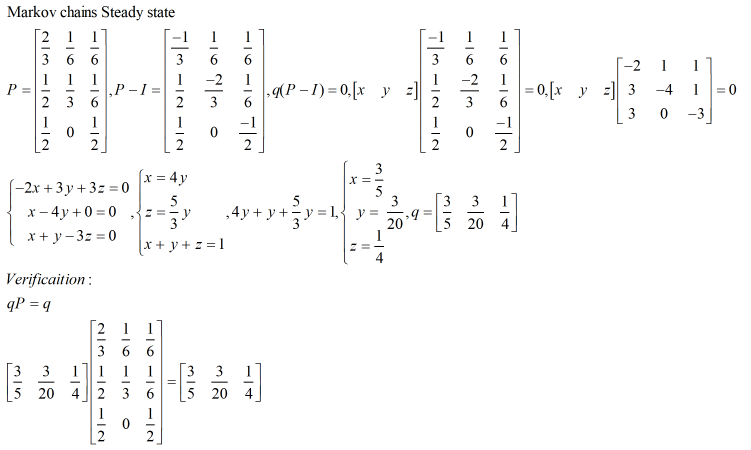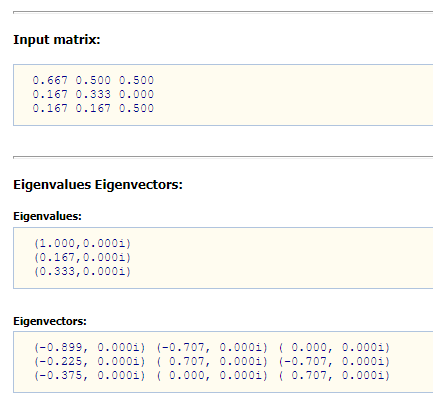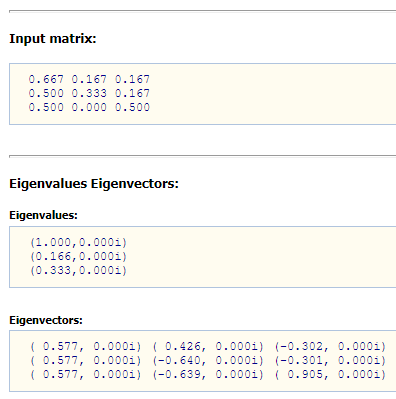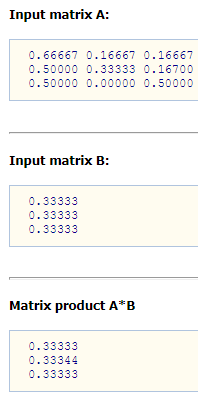A note for the eigenvector used in the Markov Steady Status: using P transpose or P to calculate eigenvector

A note for the eigenvector used in the Markov Steady Status: usingor P to calculate eigenvector?

Last night, a classmate of my friend asks a good question about the eigenvector used for the Markov Steady status. Do we use Markov probability transition matrix to calculate its eigenvalue, or use its transpose to calculate? Why?

Here is an example. The P matrix below is the Markov probability transition matrix: sum of each row probability is 1.  You can image the 3 nodes with transition graph as below:

[Image_Link]https://lh5.googleusercontent.com/51WzS0bA9iYj_89SduLR18A5IV6eBLLf6-5I86

We can compute the Markov steady status correctly as below. This is a math procedure on how to calculate the status vector S for equation SP=S.We know that the Markov transition is to calculate what the probabilities are for each node in next iteration. For example, we have 3 rooms at the beginning. We assume that a fly is stayed in the room B now. The fly will use the above matrix P to decide where it goes in next minute.

Markov probability transition problem is to calculate what the probability is for the fly in each room after next 1st minute, then 2nd minute, and finally what the convergent probability for each room after infinite minutes.

1. The simplest problem: what is the probability of the fly in each room in next 1st, 2nd minute?

This can be calculated by Total Probability Theory.

Event A0: the fly at room A at previous minute.

Event A1: the fly at room A at next minute.

Event B0: the fly at room B at previous minute.

Event B1: the fly at room B at next minute.

Event C0: the fly at room C at previous minute.

Event C1: the fly at room C at next minute.

Then we can denote A1|A0 as “the fly at room A at previous minute, then go to A1 at next minute”. Similar for B1|B0, C1|C0.

So, According to Total Probability Theory,

P(A1)=P(A1|A0)P(A0)+ P(A1|B0)P(B0)+ P(A1|C0)P(C0)

P(B1)=P(B1|A0)P(A0)+ P(B1|B0)P(B0)+ P(B1|C0)P(C0)

P(C1)=P(C1|A0)P(A0)+ P(C1|B0)P(B0)+ P(C1|C0)P(C0)

In the above 3 formula, it is the style of sum of multiplication, which is the matrix/vector multiplication style. We can consolidate the above 3 equation into 1 matrix equation as below (to make it simply, we will denote P(X) as X below)You can find out that the first vector is the previous probability status (denote as vector), the 2nd matrix is Markov probability transition matrix (denote as matrix P), and the final vector is the next probability status (denote as vector). So, we can further simply it as below:So, if you want to calculate the next probability that the fly stays in each room, you just need to multiply the previous status vectorwith the matrix P.  For example, if at the beginning the fly is in the room B, we can get the probability of the fly in 1st minute as below:It makes sense as it is same as the 2nd row of the matrix.

Ok, what is the probability of fly at each room in the 2nd minute?

That is easy, we just continue to multiply the vector and the matrix as below:We can continue to do as many as you can. So, it will bring the next question: will the S(Next) same as S(Previous) after infinite minute? If has, we called it as Markov steady status.

1. What is the steady probability of the fly in each room after infinite minute?

When handle the infinite, we has to use math formula to derive it. Actually, it is very simple as below., we need to solve the.

If you have basic Linear Algebra knowledge, you may find out it is similar to the formula when we want to calculate the eigenvalue/eigenvector of P with eigenvalue=1. But it is Different.

When we calculate the eigenvalue/ eigenvector for P, the formula is PX=X.  As many software can be used to calculate eigenvalue/ eigenvector, it will be a piece of cake to solveif our formulacan apply it.  Yes, we can do it with a minor transposition modification of the formula as below:That is the same equation when we calculate the eigenvalue/ eigenvector for. So, we can use eigenvalue/ eigenvector software to calculate our Markov steady status.  But remember, you has to useto calculate eigenvector instead of use P.

Here is the online software to calculate the eigenvector:(Assumed that we know that one of the eigenvalue for the eigenvector is 1 in the Markov probability transition matrix. This is the intrinsic characteristics of Markov probability transition matrix.)

So, the result is S=(-0.899, -0.225, -0.375). Actually, the result is a little bias from theory value as the precision of software. The theory valueAs the probability can’t be negative. Also, it must be sum to 1. So, we need to normalize the S. it is easy, first multiply -1, and then divide with the sum of the 3 elements, as below:The answer is same as the result when we calculate manually as linear equation systems.

1. If we calculate eigenvector with P for our Markov steady status, is it correct?

The answer is No. Here is the wrong Markov steady status result for the example.If you use the S=(0.577,0.577,0.577) to normalize to probability rule, you will getwhich is wrong.

Maybe you will argue that PS=S (as below diagram).  It looks like it match the Markov steady status concept.However, the mistaken here is that the P multiplies with S do NOT express our probability transition from previous status to next status. It does not express the concept of Total Probability Theory.  Below is the explanation in math:Let’s decompose the first equation in algebra style from the above matrix-style equation systems.You will find out that the equation does not comply with our Total Probability Theory. The above formula result is nonsense.   So, we can’t use P to get our eigenvector as our Markov steady probability status vector. Instead, we must use.